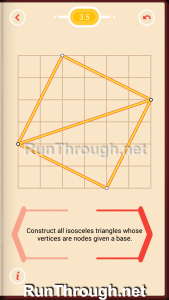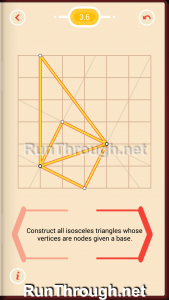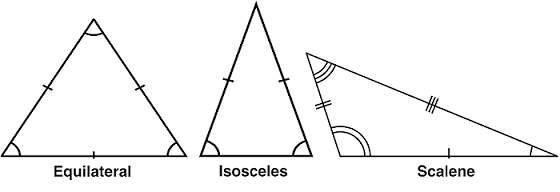# Pythagorea Set 3 Isosceles Triangles Walkthrough and Answers

Walkthrough, solutions, and answers for Pythagorea, a puzzle game in the Google Play and Apple iTunes store. This is the full solution, answer, and walkthrough for set 3, Isosceles Triangles. Follow along by downloading the app, and see the rest of the solutions at the bottom of this walkthrough!

For this walkthrough (solution, answer) for Pythagorea, the final solution will be shown.  These can be seen as the final solution as the lines or dots are glowing orange.

## Pythagorea Walkthrough Set 3, Isosceles Triangles

### Level 1### Level 2### Level 3### Level 4### Level 5### Level 6### Level 7### Level 8### Level 9### Level 10### Level 11All Sets: 1 | 2 | 3 | 4 | 5 | 6 | 7 | 8 | 9 | 10 | 11 | 12 | 13 | 14 | 15 | 16 | 17 | 18 |19 | 20 | 21 | 22 | 23 | 24 | 25 | 26

See Pythagorea Review

## Pythagorea Isosceles Triangles vs Other Triangles

There are three different types of triangles, equilateral, isosceles, and scalene.  They are defined below:

• Equilateral – All (3) sides are equal length, as well as all (3) angles are the same
• Isosceles – A triangle with at least two equal sides, as well as 2 equal angles
• Scalene – A triangle with no two sides or angles the same

As a isosceles triangle has at least two sides with the same length, an equilateral triangle is technically a isosceles triangle, but when people refer to isosceles triangles, they are usual referring to a triangle with exactly 2 equal sides and 2 equal angles.  The 2 equal angles next to the side that is the odd length out, as seen in the image below:The images shows the difference in the triangles.  The lines marking the sides and angles are showing the equal sides and angles.  The sides with the same amount of marks/lines are equal, as well as the angles with the same amount of angle markings are also equal.  For more information about the difference in triangles, check out identifying scalene, isosceles, and equilateral triangles.

This is one of two dedicated triangle level in Pythagorea.  The next one is in set 21, right triangles, so we will talk about what makes those special on that level!  Enjoy Pythagorea, and check back often for more walkthroughs and more interesting geometry facts.